Examples

Chapter 15 Class 10 Probability (Term 1)
Serial order wise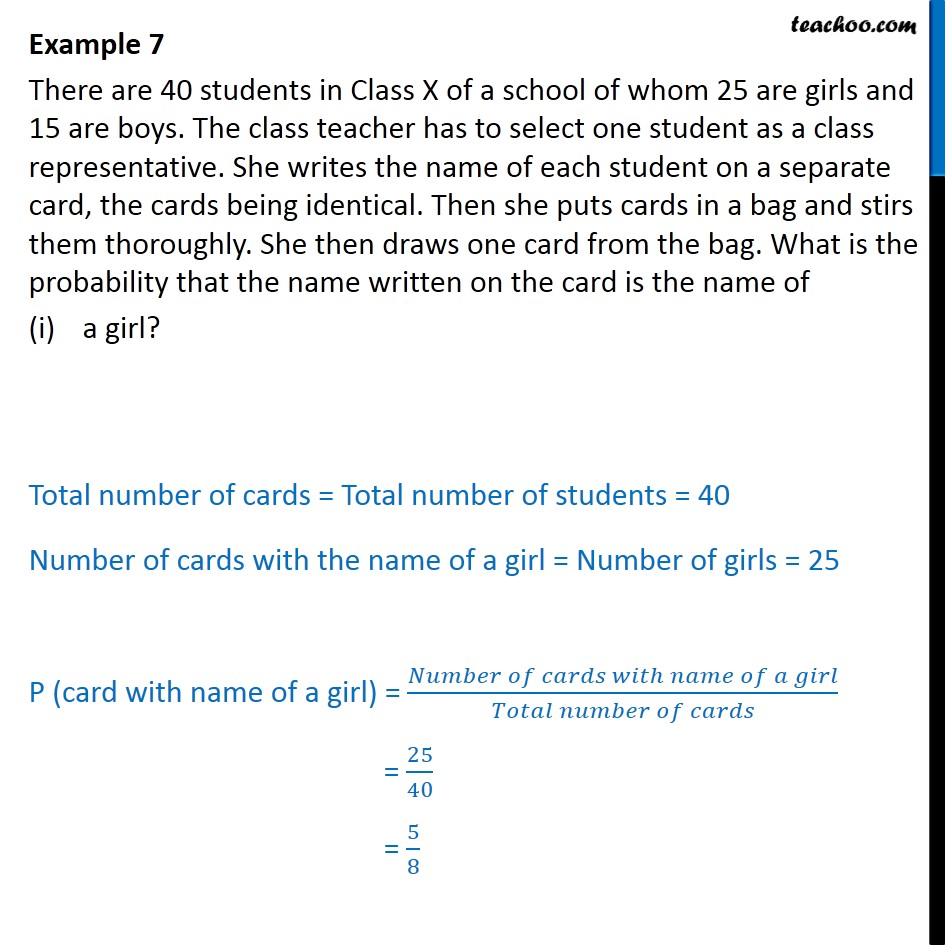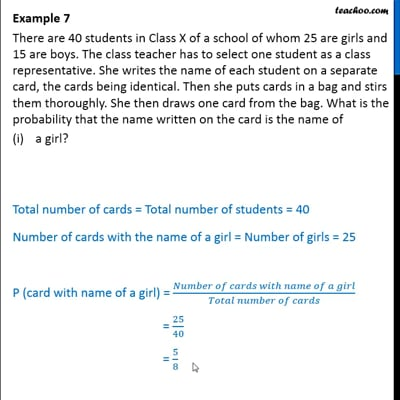This video is only available for Teachoo black users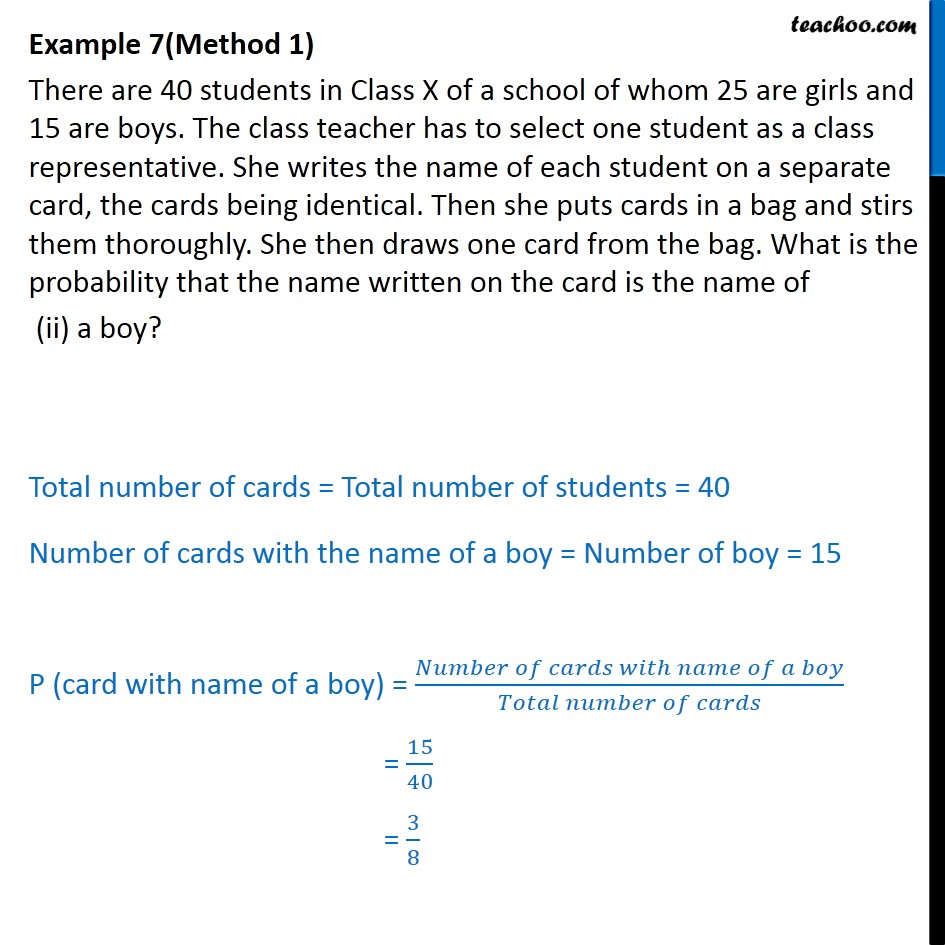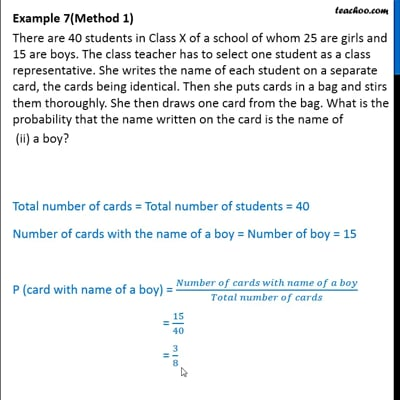This video is only available for Teachoo black users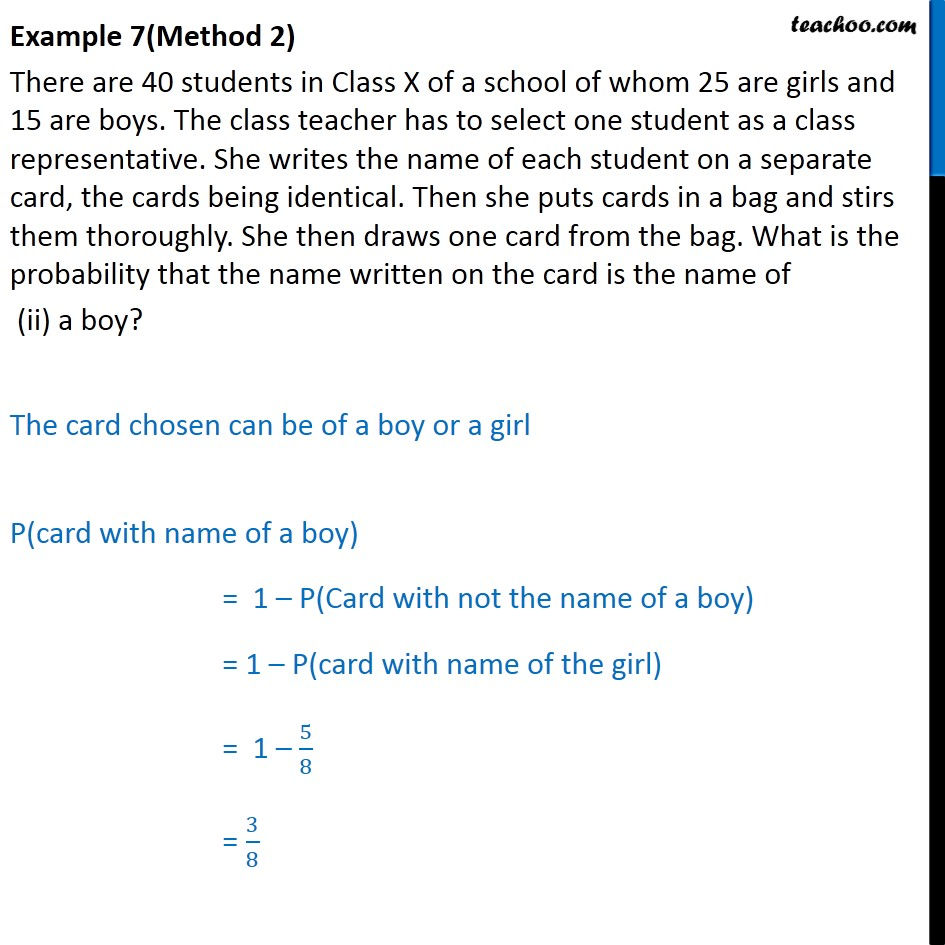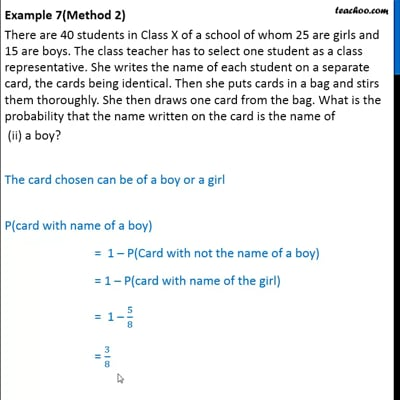This video is only available for Teachoo black users

### Transcript

Example 7 There are 40 students in Class X of a school of whom 25 are girls and 15 are boys. The class teacher has to select one student as a class representative. She writes the name of each student on a separate card, the cards being identical. Then she puts cards in a bag and stirs them thoroughly. She then draws one card from the bag. What is the probability that the name written on the card is the name of a girl? Total number of cards = Total number of students = 40 Number of cards with the name of a girl = Number of girls = 25 P (card with name of a girl) = ( )/( ) = 25/40 = 5/8 Example 7(Method 1) There are 40 students in Class X of a school of whom 25 are girls and 15 are boys. The class teacher has to select one student as a class representative. She writes the name of each student on a separate card, the cards being identical. Then she puts cards in a bag and stirs them thoroughly. She then draws one card from the bag. What is the probability that the name written on the card is the name of (ii) a boy? Total number of cards = Total number of students = 40 Number of cards with the name of a boy = Number of boy = 15 P (card with name of a boy) = ( )/( ) = 15/40 = 3/8 Example 7(Method 2) There are 40 students in Class X of a school of whom 25 are girls and 15 are boys. The class teacher has to select one student as a class representative. She writes the name of each student on a separate card, the cards being identical. Then she puts cards in a bag and stirs them thoroughly. She then draws one card from the bag. What is the probability that the name written on the card is the name of (ii) a boy? The card chosen can be of a boy or a girl P(card with name of a boy) = 1 P(Card with not the name of a boy) = 1 P(card with name of the girl) = 1 5/8 = 3/8